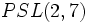# Groups of order 168

(diff) ← Older revision | Latest revision (diff) | Newer revision → (diff)

## Contents

See pages on algebraic structures of order 168| See pages on groups of a particular order

## Statistics at a glance

The prime factorization of 168 is:$\! 169 = 2^3 \cdot 3 \cdot 7 = 8 \cdot 3 \cdot 7$

Quantity Value List/comment
Total number of groups 57
Total number of abelian groups 3 ((number of abelian groups of order 8) = 3) times (number of abelian groups of order 3) = 1) times (number of abelian groups of order 7) = 1). See classification of finite abelian groups and structure theorem for finitely generated abelian groups.
Total number of nilpotent groups 5 ((number of groups of order 8) = 5) times ((number of groups of order 3) = 1) times ((number of groups of order 5) = 1). See equivalence of definitions of finite nilpotent group
Total number of solvable groups 56 the only non-solvable group is the simple non-abelian group projective special linear group:PSL(3,2), which is also isomorphic to$PSL(2,7)$.
Total number of simple groups 1 the simple non-abelian group projective special linear group:PSL(3,2), which is also isomorphic to$PSL(2,7)$.